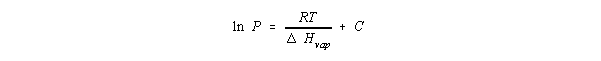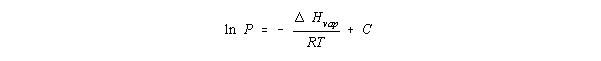The Clausius-Clapeyron Equation

The Clausius-Clapeyron Equation

The relationship between the temperature of a liquid and its vapor pressure is not a straight line. The vapor pressure of water, for example, increases significantly more rapidly than the temperature of the system. This behavior can be explained with the Clausius-Clapeyron equation.According to this equation, the rate at which the natural logarithm of the vapor pressure of a liquid changes with temperature is determined by the molar enthalpy of vaporization of the liquid, the ideal gas constant, and the temperature of the system. If we assume that Hvap does not depend on the temperature of the system, the Clausius-Clapeyron equation can be written in the following integrated form where C is a constant.This form of the Clausius-Clapeyron equation has been used to measure the enthalpy of vaporization of a liquid from plots of the natural log of its vapor pressure versus temperature. For our purposes, it would be more useful to take advantage of logarithmic mathematics to write this equation as follows.Because the molar enthalpy of vaporization of a liquid is always a positive number, this equation suggests that the logarithm of the vapor pressure will increase as the temperature of the system increases. Since the vapor pressure of the liquid increases much more rapidly than its natural logarithm, we get the behavior observed in the plot of vapor pressure versus temperature.